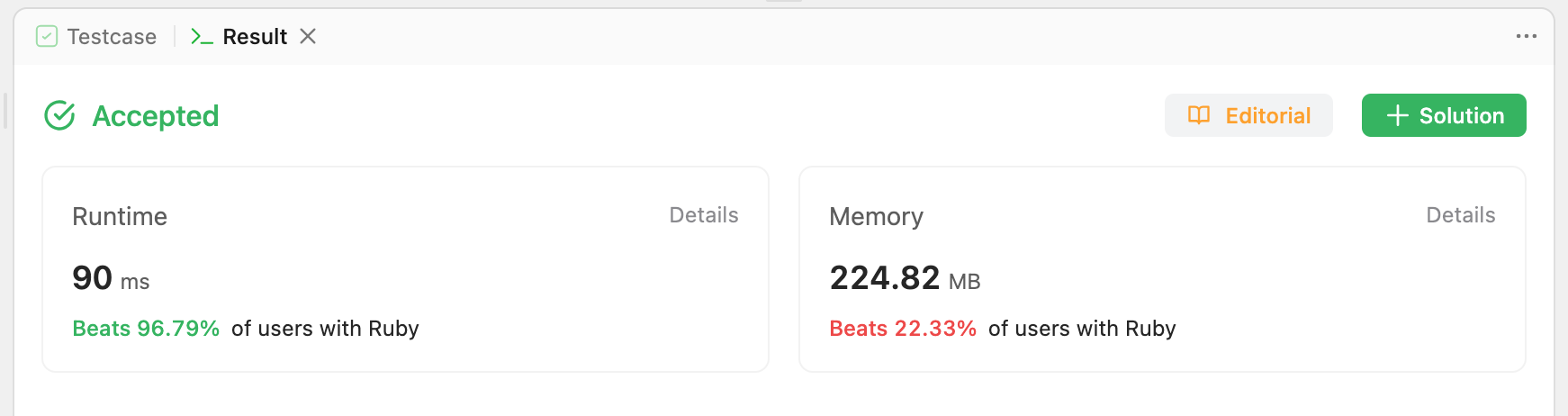Sep 26, 2023 · 1 mins readProblem Statement -

Given an integer array nums, return true if any value appears at least twice in the array, and return false if every element is distinct.

leetcode problem link - Contains Duplicate

## High level overview -

We need to return true/false if any integer appears twice in given array. Goal here is to do it in O(n) time complexity and O(n) extra space.

To check if integer has already appeared in the array, we need to store the processed values somewhere. So what could be the best choice? How will we select the data structure? The data structure that best suits our case is a Hash, because we will store integers as keys.

We will iterate through each int, and check if it is present in the Hash. If yes, then we can say that the number has appeared twice and return truthy response. If no, then make entry for this integer in Hash so that it can be found in next appearance of the same integer.

And if we reach outside the loop, then we can come to conclusion that No integer appeared twice in the array.

## Algorithm -

1. Initiate an hash to store the processed integers, initially empty
2. Iterate through each integer
3. check if this integer as key is present in the Hash - if yes, then return truthy and exit function
4. if no, then push the integer as key into hash (what to keep as value? I am going with simple boolean)
5. End loop
6. Return falsy and exit function

## Code -

``````# @param {Integer[]} nums
# @return {Boolean}
def contains_duplicate(nums)
h = {}
nums.each do |n|
return true if h.key? n

h[n] = true
end
false
end
``````

## Benchmarking -

Beats 96.79%of users with Ruby | Runtime : 90 msHappy Coding!!!